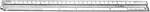### The Scale of the Architects

Scales are made with different divisions for different purposes. For machine, structural and architectural…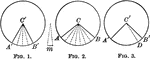### Equal Circles With Intercepted Arcs

Illustration of equal circles to show that two central angles have the same ratio as their intercepted…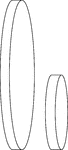### 2 Narrow Cylinders on Their Sides

Illustration of 2 narrow right circular cylinders with equal heights (thickness) and different diameters.…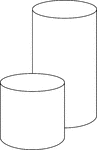### 2 Right Circular Cylinders

Illustration of 2 right circular cylinders in which one cylinder is twice the height of the other.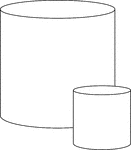### 2 Right Circular Cylinders

Illustration of 2 right circular cylinders in which one cylinder is twice the height and twice the diameter…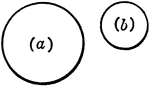### Similar Figures

2 circles that are similar figures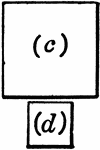### Similar Figures

2 squares that are similar figures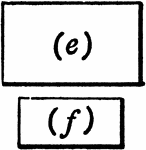### Similar Figures

2 rectangles that are similar figures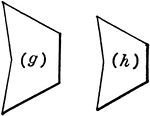### Similar Figures

2 pentagons that are similar figures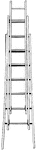Illustration of an extension ladder. If ladder is leaned against a building, it will form a right triangle…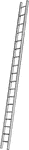Illustration of a ladder that is not perpendicular to the ground. If it is set on the ground and leaned…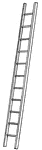Illustration of a ladder that is not perpendicular to the ground. If it is set on the ground and leaned…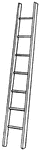Illustration of a ladder that is not perpendicular to the ground. If it is set on the ground and leaned…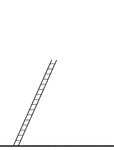Illustration of a ladder that is not perpendicular to the ground. If it is set on the ground and leaned…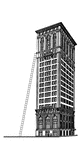### Ladder Leaning Against a Building

Illustration of a ladder leaning against the side of a building (wall) to form a right triangle .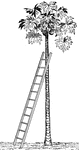### Ladder Leaning Against a Tree

Illustration of a ladder leaning against a palm tree, that is perpendicular to the ground, to form a…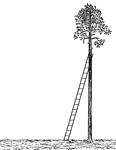### Ladder Leaning Against a Tree

Illustration of a ladder leaning against a palm tree, that is perpendicular to the ground, to form a…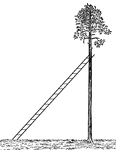### Ladder Leaning Against a Tree

Illustration of a ladder leaning against a palm tree, that is perpendicular to the ground, to form a…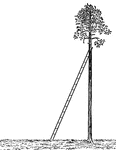### Ladder Leaning Against a Tree

Illustration of a ladder leaning against a palm tree, that is perpendicular to the ground, to form a…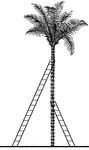### 2 Ladders Leaning Against a Tree

Illustration of 2 ladders leaning against opposite sides of a palm tree to form similar right triangles.…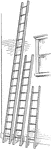### 3 Ladders Leaning Against a Wall

Illustration of 3 ladders leaning against the side of a building (wall) to form right triangles. The…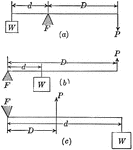### Lever and Fulcrum

Illustration of a lever with fulcrum F. W represents the weight lifted, P is the force that does the…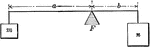### Lever Balanced on fulcrum With Weights

An illustration of a lever with weights m and n with distances a and b from fulcrum. Illustration could…### Hammer Used as a Lever

Illustration of a hammer being used as a lever. F represents the fulcrum.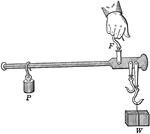### Steel-yard Used as a Lever

Illustration of an ordinary steel-yard being used as a lever. F represents the fulcrum. Weight P is…### Parallel Lines Cut by Transversals

Illustration that shows if two parallel lines are cut by three or more transversals that pass through…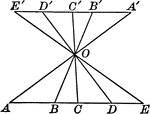### Parallel Lines Cut by Transversals

Illustration that shows if two parallel lines are cut by three or more transversals that pass through…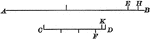### Straight Lines to Find Ratios

Illustration of two straight lines that can be used to find ratios.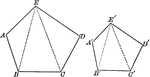### Similar Pentagons

Illustration of 2 similar pentagons.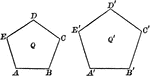### Similar Pentagons

Illustration of 2 similar pentagons.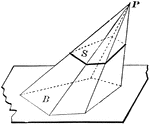### Pyramid Cut By Plane

Diagram used to prove the theorem: "If a pyramid is cut by a plane parallel to the base, the edges are…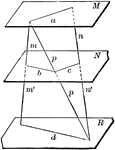### 3 Parallel Planes

"If two straight lines are cut by three parallel planes, the corresponding segments are proportional."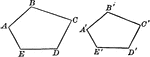### Similar Polygons (Pentagons) That Can Be Used To Show Proportionality

Illustration that shows similar polygons (pentagons) that can be used to show proportionality.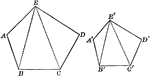### Similar Polygons (Pentagons) That Can Be Used To Show Proportionality

Illustration that shows similar polygons (pentagons) that can be used to show proportionality.### Similar Polyhedrons

Two similar polyhedrons may be decomposed into the same number of tetrahedrons similar, each to each,…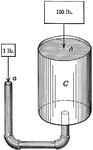### Hydraulic Machine Exerting Pressure

Illustration of a hydraulic machine. "A principle known as Pascal's Law states that pressure exerted…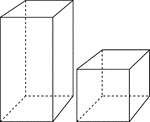### 2 Rectangular Prisms

Illustration of 2 right rectangular prisms. The bases are congruent, but the height of the smaller prism…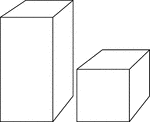### 2 Rectangular Prisms

Illustration of 2 right rectangular prisms. The bases are congruent, but the height of the smaller prism…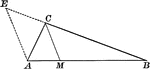### Angle Bisector of Triangle Dividing Opposite Side Into Proportionate Segments

Illustration to show that an angle bisector of a triangle divides the opposite side into segments which…### Exterior Angle Bisector of Triangle Dividing Opposite Side Into Proportionate Segments

Illustration to show that a bisector of a an exterior angle of a triangle divides the opposite side…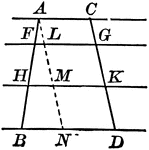### Parallel Lines Cut by Parallels, Corresponding Intercepts Proportional

Illustration to show that if parallel lines are cut by any number of parallels, the corresponding intercepts…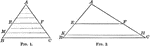### Triangle Divided by Parallel Lines to Form Proportionate Triangles

Illustration to show that if a line is drawn through two sides of a triangle parallel to the third side,…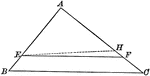### Triangle Divided by Parallel Lines to Form Proportionate Triangles

Illustration to show that if a line divides two sides of a triangle proportionally, it is parallel to…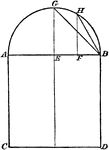### Construction of Proportional Square

Illustration used to construct a square that shall be in proportion to a given square.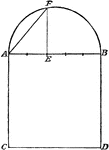### Construction of Proportional Square

Illustration used to construct a square that shall be in proportion to a given square.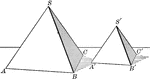### Similar Tetrahedrons

The volumes of two similar tetrahedrons are to each other as the cubes of their homologous (corresponding)…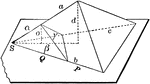### Two Proportional Tetrahedrons

Diagram used to prove the theorem: "Two tetrahedrons having a trihedral angle in each equal, are to…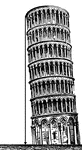### Leaning Tower

Illustration of a leaning tower with a perpendicular drawn from the top of the tower to the ground to…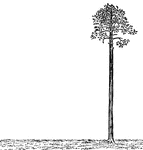### Palm Tree Perpendicular to Ground

Illustration of a palm tree that is perpendicular to the ground. The tree is perfectly straight, as…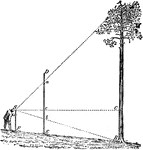### Using Proportions To Find Heights of Trees

Triangle diagram for measuring heights of trees using proportions.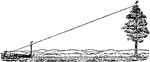### Using Proportions To Find Heights of Trees

Triangle diagram for measuring heights of trees using proportions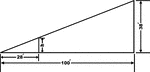### Inclined Plane Triangle

Inclined plane using right triangle and proportions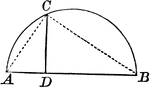### Right Triangle Inscribed in Semicircle Shows Mean Proportional

Right triangle inscribed in semicircle. Illustration shows that the perpendicular from any point in…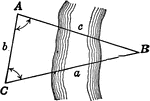### Oblique Triangle for Distance Across a River

Illustration of oblique triangle used to find distance across a river.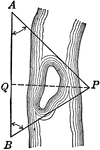### Oblique Triangle for Distance Across a River

Illustration of oblique triangle used to find distance across a river.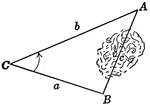### Oblique Triangle for Distance Across Lake

Illustration of oblique triangle used to find distance across a lake.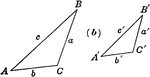### Similar Triangles

Similar triangles with corresponding sides labeled.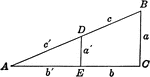### Similar Triangles

Similar triangles with corresponding sides labeled.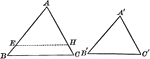### Mutually Equiangular Similar Triangles

Illustration that shows two mutually equiangular triangles are similar.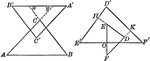### Similar Triangles With Respective Sides Parallel or Perpendicular

Illustration that shows that two triangles which have their sides respectively parallel, or respectively…